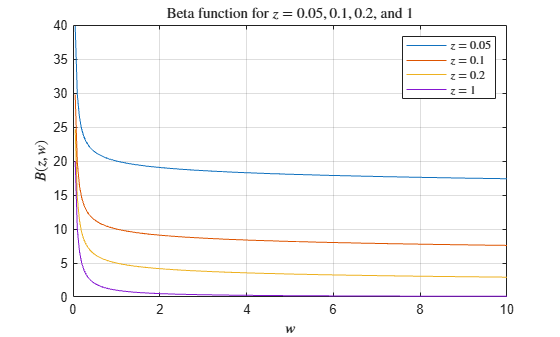# beta

## Syntax

``B = beta(Z,W)``

## Description

example

````B = beta(Z,W)` returns the beta function evaluated at the elements of `Z` and `W`. Both `Z` and `W` must be real and nonnegative.```

## Examples

collapse all

Compute the beta function for integer arguments $w=3$ and $z=1,...,10$. Based on the definition, the beta function can be calculated as

$\mathit{B}\left(\mathit{z},3\right)=\frac{\Gamma \left(\mathit{z}\right)\Gamma \left(3\right)}{\Gamma \left(\mathit{z}+3\right)}=\frac{\left(\mathit{z}-1\right)!\text{\hspace{0.17em}}2!}{\left(\mathit{z}+2\right)!}=\frac{2}{\mathit{z}\left(\mathit{z}+1\right)\left(\mathit{z}+2\right)}$.

Set the output format to rational to show the results as ratios of integers.

```format rat B = beta((1:10)',3)```
```B = 1/3 1/12 1/30 1/60 1/105 1/168 1/252 1/360 1/495 1/660 ```

Calculate the beta function for $z$ = 0.05, 0.1, 0.2, and 1 within the interval $0\le w\le 10$. Loop over values of $z$, evaluate the function at each one, and assign each result to a row of `B`.

```Z = [0.05 0.1 0.2 1]; W = 0:0.05:10; B = zeros(4,201); for i = 1:4 B(i,:) = beta(Z(i),W); end```

Plot all of the beta functions in the same figure.

```plot(W,B) grid on legend('\$z = 0.05\$','\$z = 0.1\$','\$z = 0.2\$','\$z = 1\$','interpreter','latex') title('Beta function for \$z = 0.05, 0.1, 0.2\$, and \$1\$','interpreter','latex') xlabel('\$w\$','interpreter','latex') ylabel('\$B(z,w)\$','interpreter','latex')```## Input Arguments

collapse all

Input array, specified as a scalar, vector, matrix, or multidimensional array. The elements of `Z` must be real and nonnegative. `Z` and `W` must be the same size, or else one of them must be a scalar.

Data Types: `single` | `double`

Input array, specified as a scalar, vector, matrix, or multidimensional array. The elements of `W` must be real and nonnegative. `Z` and `W` must be the same size, or else one of them must be a scalar.

• If `Z` or `W` is equal to 0, the beta function returns `Inf`.

• If `Z` and `W` are both 0, the beta function returns `NaN`.

Data Types: `single` | `double`

collapse all

### Beta Function

The beta function is defined by

`$B\left(z,w\right)={\int }_{0}^{1}{t}^{z-1}{\left(1-t\right)}^{w-1}dt=\frac{\Gamma \left(z\right)\Gamma \left(w\right)}{\Gamma \left(z+w\right)}.$`

The $\Gamma \left(z\right)$ term is the gamma function

`$\Gamma \left(z\right)={\int }_{0}^{\infty }{t}^{z-1}{e}^{-t}dt.$`

 Olver, F. W. J., A. B. Olde Daalhuis, D. W. Lozier, B. I. Schneider, R. F. Boisvert, C. W. Clark, B. R. Miller, and B. V. Saunders, eds., Chapter 5.12 Beta Function, NIST Digital Library of Mathematical Functions, Release 1.0.22, Mar. 15, 2018.